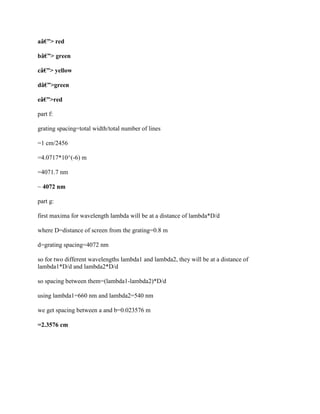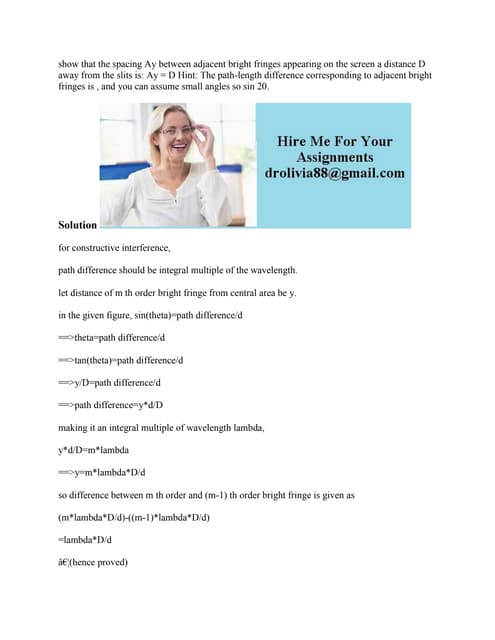AnúncioPróximos SlideSharesshow that the spacing Ay between adjacent bright fringes appearing on.docx
Carregando em ... 3
1 de 3
Anúncio

### Please show all work- Thanks- (I believe the diagram is marked 80cm fo.docx

1. Please show all work. Thanks. (I believe the diagram is marked 80cm for the distances of a, b, c, and d...) HY162 Exam 4 ay 2017 Name (20 pts) A two color laser emits a single beam of light that is a mixture of two different colors, 660 to our eye. 1. nm red and 540 nm green. These two colors mixed together appear yellow 80.6 cm two color lasex mixed bee m ?. qrati Scceen The laser beam passes through a transmission diffraction grating as shown. What are the colors (red, green, or yellow) of the spots on the screen? Spots are labeled as follows: a) b) c) d) e) f) if the diffraction grating has 2456 lines in 1.000 cm of width, show that the grating spacing is d = 4072 nm. g) What is the distance on the screen between points a and b? Solution for diffraction grating, d*sin(theta)=m*lambda where d=grating spacing theta=angle with the normal m=order of maximum lambda=wavelength so higher the wavelength, higher will be the distance from central spot. at the central spot, both the color will have a central bright fringe and hence their combination will appear as yellow. with green having lesser wavelength, it will have less distance from the central spot hence point b and d will be green and point a and e will be red. hence answers are:
2. aâ€”> red bâ€”> green câ€”> yellow dâ€”>green eâ€”>red part f: grating spacing=total width/total number of lines =1 cm/2456 =4.0717*10^(-6) m =4071.7 nm ~ 4072 nm part g: first maxima for wavelength lambda will be at a distance of lambda*D/d where D=distance of screen from the grating=0.8 m d=grating spacing=4072 nm so for two different wavelengths lambda1 and lambda2, they will be at a distance of lambda1*D/d and lambda2*D/d so spacing between them=(lambda1-lambda2)*D/d using lambda1=660 nm and lambda2=540 nm we get spacing between a and b=0.023576 m =2.3576 cm
Anúncio